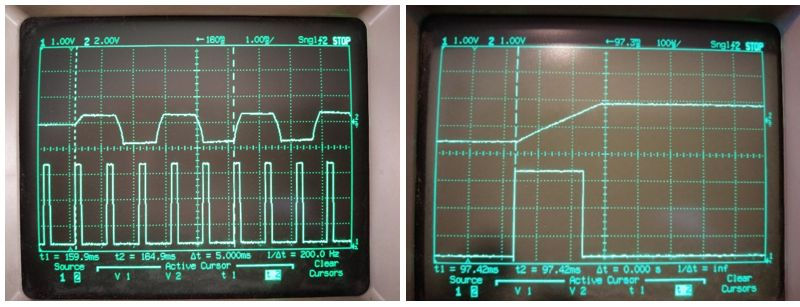top of page

SINSIN is a mathematical method, developed in the course of the SINSIN GSA project, implemented in software at the beacon and MEOLUT, enhancing the the localization availability and accuracy of the SAR/Galileo service.

SINSIN is particularly effective in terrestrial SAR sites, where only a limited number of satellites are in view with the beacon, due to obstruction of mountains, trees, buildings, etc.

With 1-4 satellites in view, SINSIN improves the localization accuracy by x2, i.e. reducing 75% of searching time, on average.

SINSIN is comprised of three sub-methods: SINSIN-a, SINSIN-b, SINSIN-c, each effective at different conditions, such as the number of satellites in view and whether UTC is acquired.

SINSIN-a

• The beacon is configured to transmit successive 406MHz bursts at a Difference in Time of Emission (DTOE) of n*ms, where n is an integer number dynamically selected in a pseudo-random pattern at the beacon, however unknown at the detector.

• The Detector (on-scene DF or SAR payload reporting to MEOLUT) measuring the Difference in Time of Arrival (DTOA) of the 406MHz bursts, while moving between a first point and a second point, can determine the following equation (see fig.1):

c*[(DTOA – DTOE) - n*ms)] = S2 – S1; wherein c is the speed of light, and S1 (distance         between beacon and first point) and S2 (distance between beacon and second point)         can each be expressed in a Pythagorean equation using the detector (known)                       coordinates at said two points and the beacon (unknown) coordinates.​

• Further the detector, assigning PR= c*(DTOA – DTOE), can determine:

[eq.1]: PR - n*300Km = S2 – S1.

Hence, eq.1 defines a hyperbola (curve of points with constant difference in distance         to two other points=foci), on which the beacon is placed, where the DTOA measuring         points are the hyperbola foci, and hyperbola major axis (S2 – S1) with ambiguity of             n*300Km.SINSIN-b

• GNSS satellites broadcast a PRN (spreading) code cyclically every 1ms, after an initial epoch txe; the epoch determining the phase of the GNSS clock; the GNSS clock acquirable at any GNSS receiver, anywhere.

• The beacon is configured to select one of the detected GNSS PRN codes and transmit a 406MHz burst T1 after detecting this GNSS PRN code; we assume that the beacon selects a GNSS code broadcast at (txe + n*1ms) [GPS time], and T1 configured as p*1ms, where n and p are integer numbers unknown at the detector.

• The Detector (on-scene DF or SAR satellite reporting to MEOLUT) measuring the Time of Arrival (TOA) of the 406MHz burst, and acquiring txe from the GNSS, can determine the following equation (see fig.2):

• c*[TOA – (txe + n*1ms) - p*1ms] = S1 + S2; wherein c is the speed of light, and S1 (distance between beacon and GNSS satellite) and S2 (distance between beacon and detector) can each be expressed in a Pythagorean equation using the satellite (known) and detector (known) and beacon (unknown) coordinates.

• Further the detector, assigning k= (n+p), and PR= c*(TOA – txe), can determine:

[eq.2]: PR - k*300Km = S1 + S2.

Hence, eq.2 defines an ellipse (curve of points with constant sum of distances to two         other points=foci), on which the beacon is placed, where the GNSS satellite and                   detector are the ellipse foci, and ellipse major axis of (S1 + S2) with ambiguity of                 k*300Km.SINSIN-c

• GNSSs enable a GNSS receiver, anywhere, determining a precise time, such as GPS Time or UTC (Universal Time Coordinated), upon acquiring four GNSS satellites and resolving the navigation equations.

• The beacon is configured to acquire UTC (or GPS Time, leap seconds are indifferent) and transmit a 406MHz burst n*ms after the rising edge of the UTC 1PPS (1Hz) clock, where n is integer, say at (txe + n*1ms) [GPS time], while at the detector txe is acquirable but n is unknown.

• The Detector (on-scene DF or SAR satellite reporting to MEOLUT) measuring the Time of Arrival (TOA) of the 406MHz burst, and acquiring txe from the GNSS, can determine the following equation (see fig.3):

c*[TOA – (txe + n*1ms)] = S2; where c is the speed of light, and S2 (distance between         beacon and detector) can be expressed in a Pythagorean equation using the                        detector (known) and beacon (unknown) coordinates.

• Further the detector, assigning PR= c*(TOA – txe), can determine:

[eq.3]: PR - n*300Km = S2.

Hence, eq.3 defines a circle (curve of points with constant distance to one other                 point), on which the beacon is placed, where the detector is at the center of this                 circle and the circle radius (S2) has an ambiguity of n*300Km.bottom of page